# Population Downscaling Using Areal Interpolation - A Comparative Analysis

## Introduction

Areal Interpolation may be defined as the process of transforming data reported over a set of spatial units (source) to another (target). Its application to population data has attracted considerable attention during the last few decades. A massive amount of methods have been reported in the scientific literature. Most of them focus on the improvement of the accuracy by using more sophisticated techniques rather than developing standardized methods. As a result, only a few implementation tools exists within the R community.

One of the most common, easy and straightforward methods of Areal Interpolation is Areal Weighting Interpolation (AWI). AWI proportionately interpolates the population values of the source features based on areal (or spatial) weights calculated by the area of intersection between the source and the target zones.

sf and areal packages provide Areal Interpolation functionality within the R ecosystem. Both packages implement (AWI). sf functionality comes up with extensive and intensive interpolation options and calculates the areal weights based on the total area of the source features (total weights). sf functionality is suitable for completely overlapping data. areal extends the existing functionality of the sf package by introducing an additional formula for data without complete overlap. In this case weights are calculated using the sum of the remaining source areas after the intersection (sum weights).

When the case involves Areal Interpolation of urban population data (small scale applications) where the source features (such as city blocks or census tracts) are somehow larger than target features (such as buildings) in terms of footprint area the sf functionality (total weights) is unable to calculate areal weights properly and therefore, is not ideal for such applications. areal functionality may be confusing for novice R (or GIS) users as it is not obvious that the weight option should be set to sum to calculate areal weights correctly.

To overcome these limitations populR is introduced. populR is suitable for Areal Interpolation of urban population and provides an AWI approach that matches the existing functionality of areal using sum weights and additionally, proposes a VWI approach which, to our knowledge, extends the existing Areal Interpolation functionality within the R ecosystem. VWI uses the area of intersection between source and target features multiplied by the building height or number of floors (volume) to guide the interpolation process.

In this vignette a comparative analysis of Areal Interpolation alternatives within the programming environment of R is carried out. sf, areal and populR results are obtained and further compared to a more realistic population distribution.

## Case Study

A small part of the city of Mytilini, Lesvos, Greece was chosen as the case study (figure below).The study area consists of 9 city blocks (source) counting 911 residents and 179 buildings units (target) including floor number information. These data are included in populR package for further experimentation.


# attach library
library(populR)

data('src')
data('trg')

source <- src
target <- trg

# plot data
plot(source['geometry'], col = "#634B56", border = NA)
plot(target['geometry'], col = "#FD8D3C", add = T)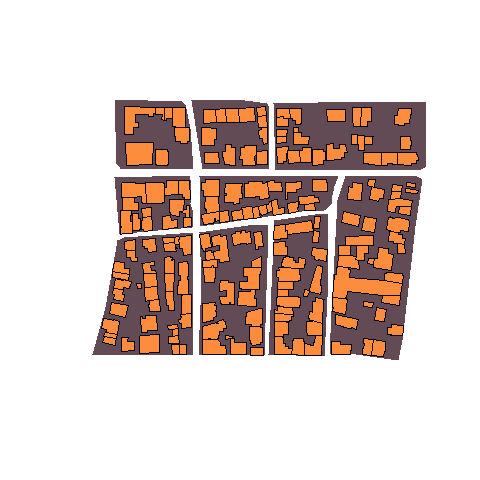## Implementation

In this section a demonstration of the sf, areal and populR packages takes place. First, the packages are attached to the script and next populR built-in data are loaded. Then, Areal Interpolation functions are executed for each one of the aforementioned packages.

The st_interpolate_aw() function of the sf package takes:

1. x: an object of class sf with data to be interpolated
2. to: the target geometries (sf object)
3. extensive: whether to use extensive (TRUE) or intensive interpolation (FALSE)

areal provides the aw_interpolate() function which requires:

1. data: an sf object to be used as target
2. tid: target identification numbers
3. source: an sf object with data to be interpolated
4. sid: source identification numbers
5. weight: may be either sum or total for extensive interpolation and sum intensive interpolation
6. output: whether sf object or tibble
7. extensive: a vector of quoted (extensive) variable names - optional if intensive is specified
8. intensive: a vector of quoted (intensive) variable names - optional if extensive is specified

Finally, populR offers pp_estimate() function which takes:

1. target: an sf object to be used as target
2. source: an sf object with data to be interpolated
3. sid: source identification number
4. spop: source population values to be interpolated
5. volume: target volume information (number of floors or height) - required for the vwi approach
6. point: whether to return point geometries (TRUE) or not (FALSE) - optional
7. method: whether to use awi or vwi

Evidently, sf package’s st_interpolate_aw function requires only 3 arguments which make it very easy to implement while populR requires at least 5 and areal at least 7 arguments which potentially increases the implementation complexity.

On the other hand, only areal may be used for multiple interpolations at once as the extensive or intensive argument takes a vector of quoted values (not included in this vignette).

For the reader’s convenience names were shortened as follows:

• awi: populR awi approach
• vwi: populR vwi approach
• aws: areal using extensive interpolation and sum weights
• awt: areal using extensive interpolation and total weights
• sf: sf using extensive interpolation

# attach libraries
library(populR)
library(areal)
library(sf)

data('src')
data('trg')

source <- src
target <- trg

# populR - awi
awi <- pp_estimate(target = target, source = source, spop = pop, sid = sid,
method = awi)
# populR - vwi
vwi <- pp_estimate(target = target, source = source, spop = pop, sid = sid,
volume = floors, method = vwi)

# areal - sum weights
aws <- aw_interpolate(target, tid = tid, source = source, sid = 'sid',
weight = 'sum', output = 'sf', extensive = 'pop')
# areal - total weights
awt <- aw_interpolate(target, tid = tid, source = source, sid = 'sid',
weight = 'total', output = 'sf', extensive = 'pop')

# sf - total weights
sf <- st_interpolate_aw(source['pop'], target, extensive = TRUE)

## Results

The study area counts 911 residents as already mentioned in previous section. From the code chunk below it is clear that awi, vwi and aws correctly estimated population values as they sum to 911 while awt and sf results underestimated values. This is expected as both methods use the total area of the source features during the interpolation process and are useful when source and target features completely overlap.


# sum initial values
sum(source$pop) #>  911 # populR - awi sum(awi$pp_est)
#>  911

# populR - vwi
sum(vwi$pp_est) #>  911 # areal - awt sum(awt$pop)
#>  412.1597

# areal - aws
sum(aws$pop) #>  911 # sf sum(sf$pop)
#>  412.1597

Moreover, identical results were obtained by the awi and aws approaches and somehow different results by the vwi as shown in the code block below.


# order values using tid
awi <- awi[order(awi$tid),] vwi <- vwi[order(vwi$tid),]

# get values and create a df
awi_values <- awi$pp_est vwi_values <- vwi$pp_est

awt_values <- awt$pop aws_values <- aws$pop

sf_values <- sf$pop df <- data.frame(vwi = vwi_values, awi = awi_values, aws = aws_values, awt = awt_values, sf = sf_values) df[1:20,] #> vwi awi aws awt sf #> 1 0.3727930 1.3583401 1.3583401 0.5764607 0.5764607 #> 2 0.3900385 1.4211775 1.4211775 0.6031280 0.6031280 #> 3 15.4717046 16.1218368 16.1218368 5.9862902 5.9862902 #> 4 4.3006948 7.4690218 7.4690218 2.7733646 2.7733646 #> 5 0.6080431 2.2155174 2.2155174 0.9402349 0.9402349 #> 6 28.3192357 21.0780217 21.0780217 7.8265992 7.8265992 #> 7 0.4389792 1.3401180 1.3401180 0.6160096 0.6160096 #> 8 1.4548990 2.5695894 2.5695894 1.3560349 1.3560349 #> 9 5.0290657 6.5358992 6.5358992 2.7491621 2.7491621 #> 10 3.8438283 6.6755812 6.6755812 2.4787477 2.4787477 #> 11 3.2144283 4.1775512 4.1775512 1.7571822 1.7571822 #> 12 1.4377011 1.6928100 1.6928100 0.8933371 0.8933371 #> 13 0.2784513 1.0145888 1.0145888 0.4305774 0.4305774 #> 14 3.3974766 4.1264445 4.1264445 1.7512059 1.7512059 #> 15 0.1030070 0.3144603 0.3144603 0.1445474 0.1445474 #> 16 1.5219709 1.7920328 1.7920328 0.9456994 0.9456994 #> 17 20.4179677 11.6472800 11.6472800 5.3922672 5.3922672 #> 18 5.1720468 8.8510686 8.8510686 4.0977230 4.0977230 #> 19 1.5706508 5.3757975 5.3757975 2.4887988 2.4887988 #> 20 8.8823230 5.1978924 5.1978924 1.7522985 1.7522985 ### Comparison to Reference Data Due to confidentiality concerns, population data at building level are not available in Greece. Therefore, an alternate population distribution previously published in Batsaris et al. 2019 was used as reference data set to compare the results. This reference population values are included in the built-in data set as shown below in the field rf.  target #> Simple feature collection with 179 features and 3 fields #> Geometry type: POLYGON #> Dimension: XY #> Bounding box: xmin: 720385 ymin: 4330206 xmax: 720645.6 ymax: 4330412 #> Projected CRS: GGRS87 / Greek Grid #> First 10 features: #> tid floors rf geometry #> 1 1 1 0.4644686 POLYGON ((720643.2 4330345,... #> 2 2 1 0.4859551 POLYGON ((720645 4330340, 7... #> 3 3 5 16.2015950 POLYGON ((720428.1 4330404,... #> 4 4 3 5.6294795 POLYGON ((720457.5 4330383,... #> 5 5 1 0.7575704 POLYGON ((720634.4 4330298,... #> 6 6 7 31.7734490 POLYGON ((720405.8 4330363,... #> 7 7 1 0.4896089 POLYGON ((720423.5 4330211,... #> 8 8 2 1.5688688 POLYGON ((720492.9 4330318,... #> 9 9 3 3.7516581 POLYGON ((720512.4 4330375,... #> 10 10 3 5.0314551 POLYGON ((720439.2 4330375,... In the code chunk below the first 20 features are presented for comparison. rf <- awi$rf

df <- cbind(rf, df)

df[1:20,]
#>            rf        vwi        awi        aws       awt        sf
#> 1   0.4644686  0.3727930  1.3583401  1.3583401 0.5764607 0.5764607
#> 2   0.4859551  0.3900385  1.4211775  1.4211775 0.6031280 0.6031280
#> 3  16.2015950 15.4717046 16.1218368 16.1218368 5.9862902 5.9862902
#> 4   5.6294795  4.3006948  7.4690218  7.4690218 2.7733646 2.7733646
#> 5   0.7575704  0.6080431  2.2155174  2.2155174 0.9402349 0.9402349
#> 6  31.7734490 28.3192357 21.0780217 21.0780217 7.8265992 7.8265992
#> 7   0.4896089  0.4389792  1.3401180  1.3401180 0.6160096 0.6160096
#> 8   1.5688688  1.4548990  2.5695894  2.5695894 1.3560349 1.3560349
#> 9   3.7516581  5.0290657  6.5358992  6.5358992 2.7491621 2.7491621
#> 10  5.0314551  3.8438283  6.6755812  6.6755812 2.4787477 2.4787477
#> 11  3.5969214  3.2144283  4.1775512  4.1775512 1.7571822 1.7571822
#> 12  1.5503237  1.4377011  1.6928100  1.6928100 0.8933371 0.8933371
#> 13  0.3469268  0.2784513  1.0145888  1.0145888 0.4305774 0.4305774
#> 14  0.0000000  3.3974766  4.1264445  4.1264445 1.7512059 1.7512059
#> 15  0.0000000  0.1030070  0.3144603  0.3144603 0.1445474 0.1445474
#> 16  1.6411948  1.5219709  1.7920328  1.7920328 0.9456994 0.9456994
#> 17 19.7122964 20.4179677 11.6472800 11.6472800 5.3922672 5.3922672
#> 18  5.9919531  5.1720468  8.8510686  8.8510686 4.0977230 4.0977230
#> 19  1.8196405  1.5706508  5.3757975  5.3757975 2.4887988 2.4887988
#> 20  9.2134815  8.8823230  5.1978924  5.1978924 1.7522985 1.7522985

populR provides a function (pp_compare()) to compare the results with alternate population data. pp_compare() produces scatter diagram, linear regression model, correlation coeficient ($$R^2$$), MAE (Mean Absolute Error) and RMSE (Root Mean Squared Error) to investigate the relationship of the results with the reference (or other) data.

Generally, the diagrams suggest strong and positive relationships in all cases. However, vwi provides the strongest relationship and $$R^2$$ coefficient. vwi provides the smallest MAE value in comparison with the other methods as shown below.


awi_error <- pp_compare(df, estimated = awi, actual = rf, title = "awi vs actual")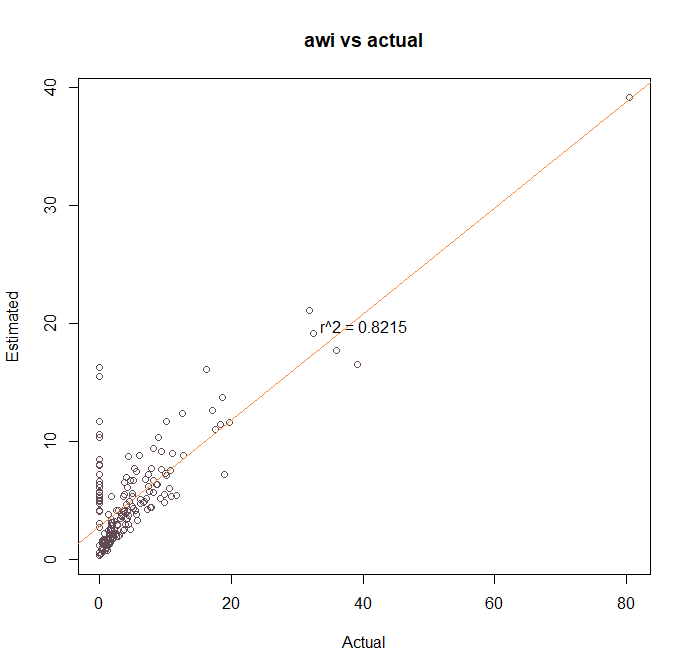awi_error
#> $rmse #>  5.325914 #> #>$mae
#>  2.748126
#>
#> $linear_model #> #> Call: #> lm(formula = x[, estimated, drop = T] ~ x[, actual, drop = T]) #> #> Coefficients: #> (Intercept) x[, actual, drop = T] #> 2.7977 0.4503 #> #> #>$correlation_coef
#>  0.8215

vwi_error <- pp_compare(df, estimated = vwi, actual = rf, title = "vwi vs actual")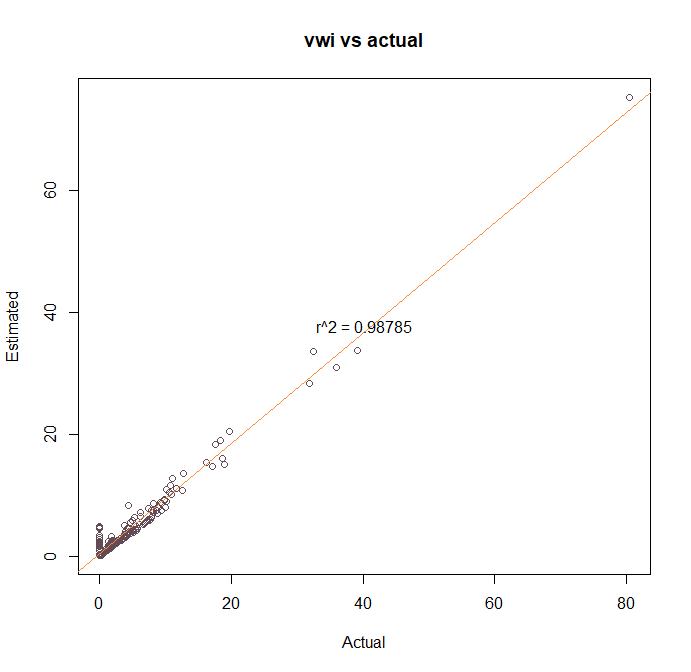vwi_error
#> $rmse #>  1.44824 #> #>$mae
#>  0.9358159
#>
#> $linear_model #> #> Call: #> lm(formula = x[, estimated, drop = T] ~ x[, actual, drop = T]) #> #> Coefficients: #> (Intercept) x[, actual, drop = T] #> 0.4926 0.9032 #> #> #>$correlation_coef
#>  0.98785

sf_error <- pp_compare(df, estimated = sf, actual = rf, title = "sf vs actual")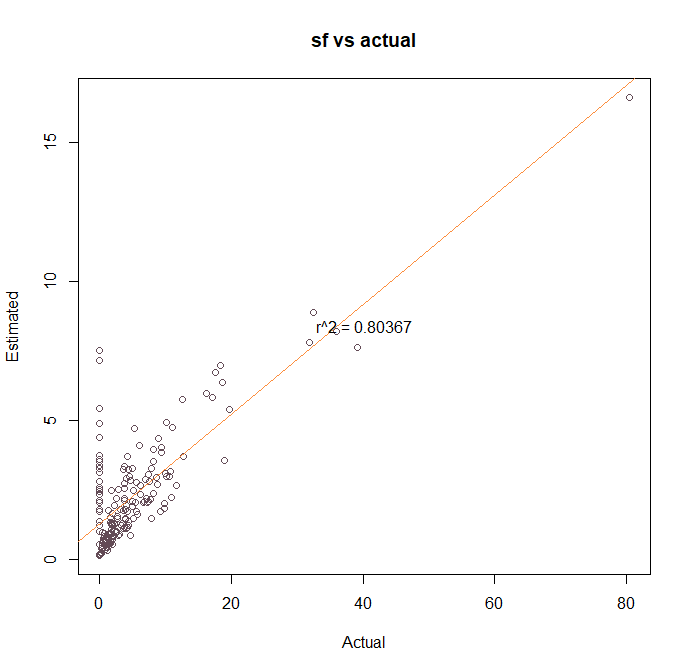sf_error
#> $rmse #>  7.416329 #> #>$mae
#>  3.664695
#>
#> $linear_model #> #> Call: #> lm(formula = x[, estimated, drop = T] ~ x[, actual, drop = T]) #> #> Coefficients: #> (Intercept) x[, actual, drop = T] #> 1.2992 0.1972 #> #> #>$correlation_coef
#>  0.80367

awt_error <- pp_compare(df, estimated = awt, actual = rf, title = "awt vs actual")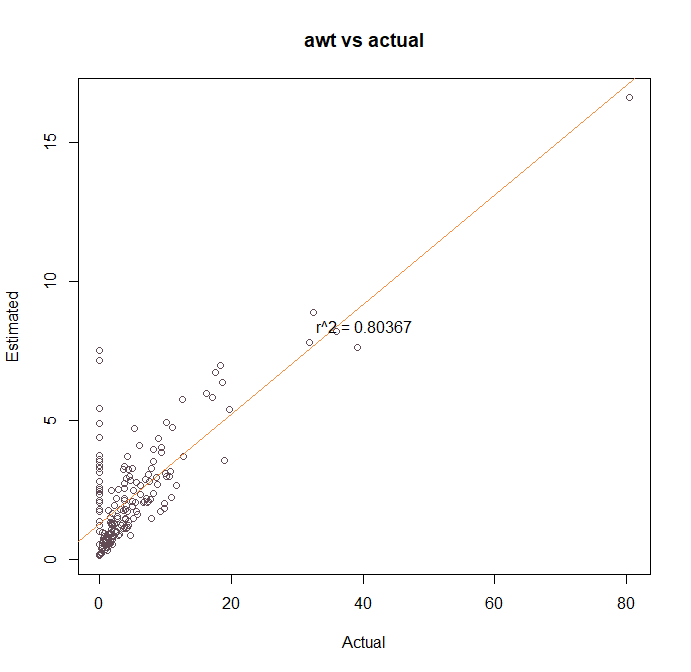awt_error
#> $rmse #>  7.416329 #> #>$mae
#>  3.664695
#>
#> $linear_model #> #> Call: #> lm(formula = x[, estimated, drop = T] ~ x[, actual, drop = T]) #> #> Coefficients: #> (Intercept) x[, actual, drop = T] #> 1.2992 0.1972 #> #> #>$correlation_coef
#>  0.80367

aws_error <- pp_compare(df, estimated = aws, actual = rf, title = "aws vs actual")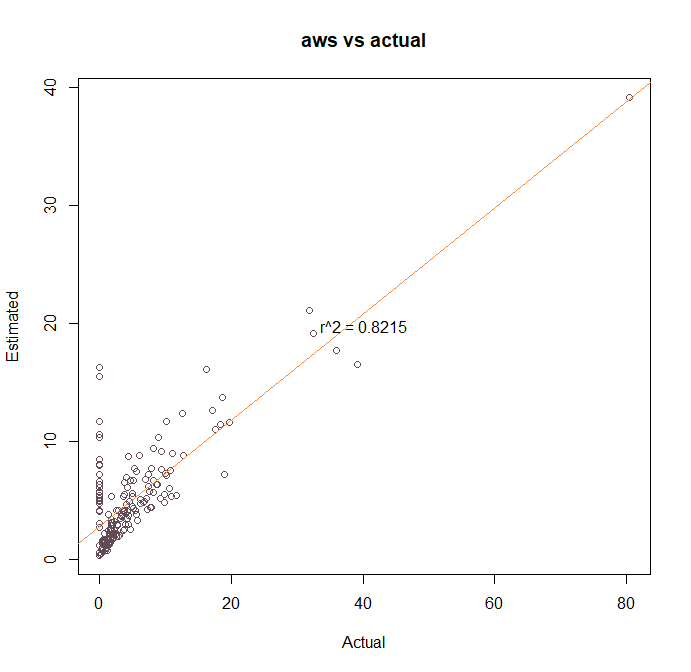aws_error
#> $rmse #>  5.325914 #> #>$mae
#>  2.748126
#>
#> $linear_model #> #> Call: #> lm(formula = x[, estimated, drop = T] ~ x[, actual, drop = T]) #> #> Coefficients: #> (Intercept) x[, actual, drop = T] #> 2.7977 0.4503 #> #> #>$correlation_coef
#>  0.8215

RMSE (Root Mean Squared Error) is also calculated. Again, vwi provides the smallest error value as shown in the code block below.

## Comparison on Performance

Finally, a performance comparison (execution times) is carried out in this section using microbenchmark package. Execution time measurements suggest that populR functionality executed much faster than areal and sf as shown below. Both awi and vwi achieved the best mean execution time (about 76.74 milliseconds). aws follows with 136.67 milliseconds and finally, awt with 180.53 milliseconds.


library(microbenchmark)
#> Warning: package 'microbenchmark' was built under R version 4.1.3

# performance comparison
microbenchmark(
suppressWarnings(pp_estimate(target = target, source = source, spop = pop, sid = sid,
method = awi)),
suppressWarnings(pp_estimate(target = target, source = source, spop = pop, sid = sid,
volume = floors, method = vwi)),
aw_interpolate(target, tid = tid, source = source, sid = 'sid',
weight = 'sum', output = 'sf', extensive = 'pop'),
aw_interpolate(target, tid = tid, source = source, sid = 'sid',
weight = 'total', output = 'sf', extensive = 'pop'),
suppressWarnings(st_interpolate_aw(source['pop'], target, extensive = TRUE))
)
#> Unit: milliseconds
#>                                                                                                                        expr
#>                   suppressWarnings(pp_estimate(target = target, source = source,      spop = pop, sid = sid, method = awi))
#>  suppressWarnings(pp_estimate(target = target, source = source,      spop = pop, sid = sid, volume = floors, method = vwi))
#>      aw_interpolate(target, tid = tid, source = source, sid = "sid",      weight = "sum", output = "sf", extensive = "pop")
#>    aw_interpolate(target, tid = tid, source = source, sid = "sid",      weight = "total", output = "sf", extensive = "pop")
#>                                                suppressWarnings(st_interpolate_aw(source["pop"], target, extensive = TRUE))
#>       min        lq      mean   median        uq      max neval
#>   71.7053  72.80210  75.15038  73.5686  77.29545  84.6436   100
#>   72.0135  72.78705  75.44242  73.8512  76.75860 124.9136   100
#>  124.5888 126.82265 130.29592 128.9688 131.99280 180.1855   100
#>  166.6036 169.89750 173.48675 172.6257 174.80065 189.4912   100
#>  131.8874 134.94315 138.33501 137.7540 139.98630 152.7998   100

## Summary

In this vignette a demonstration and a comparative analysis of areal interpolation packages implemented in urban population data is undertaken. sf and areal provide general purpose AWI functionality while populR focuses on areal interpolation of population data. Additionally, populR provides VWI which, to the best of my knowledge, extends R’s existing functionality.

Mytilini, Greece was used as the case study to investigate three main pillars: a) implementation, b) results, c) performance. Notes on implementation indicate that sf requires only 3 arguments to use while populR at least 5 and areal 7. The results provide insight that sf and awt may not be ideal for data that are not completely overlapping. Moreover, aws and awi obtained the same results while vwi outperformed the others in comparison to the reference data set. Finally, populR performs much faster than sf and areal packages.